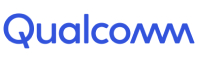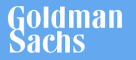New update is available. Click here to update.
Last Updated: 25 Jan, 2022

# Set Matrix Zeros

Easy+7 more companies

## Problem statement#### For Example:

``````If the given grid is this:
[7, 19, 3]
[4, 21, 0]

Then the modified grid will be:
[7, 19, 0]
[0, 0,  0]
``````

#### Input Format:

``````The first line of the input contains a single integer ‘T’ representing the no. of test cases.

The first line of each test case contains two space-separated integers ‘N’ and ‘M’, denoting the no. of the rows and columns of the matrix.

The next 'N' lines will contain ‘M’ space separated integers representing the elements of the matrix.
``````

#### Output Format:

``````For each test case, print the modified grid.

Print output of each test case in a separate line.
``````

#### Note:

``````You are not required to print anything; it has already been taken care of. Just implement the function and return the answer.
``````

#### Constraints:

``````1 ≤ T ≤ 1000
1 ≤ m, n ≤ 1000
Σ(m * n) ≤ 2000000
-2^(31) ≤ matrix[i][j] ≤ 2^(31)-1, for all (1 ≤ i ≤ n and 1 ≤ j ≤ m).

Time Limit: 1 sec
``````

``````Can we do better than O(m * n) space?
Using O(m + n) space is an improvement but we can still do better.
We can do it using constant memory. Can you do it?
``````## Approaches

### 01 ApproachThe basic idea is to maintain another boolean matrix ‘isZero’ which stores whether our resultant matrix should contain a zero at a particular index or not. We can traverse every element of our original matrix. If the element is 0, then we traverse the complete row and complete column of that particular element and set isZero values accordingly to true for all the elements in that row and column.

#### Algorithm:

setZeros(matrix)

• Store dimensions of the matrix in n and m
• Declare “isZero” boolean matrix of size n x m.
• Iterate over i from 0 to n
• Iterate over j from 0 to m
• If matrix[i][j] == 0
• Iterate over k from 0 to n
• Set isZero[k][j] = true
• Iterate over k from 0 to m
• Set isZero[i][k] = true
• Iterate over i from 0 to n
• Iterate over j from 0 to m
• If isZero[i][j] == true
• Set matrix[i][j] = 0